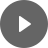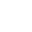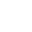5.2 Ratios - StudyNinja
Select Page

HSC Standard Maths Resources

Browse: 1. Home  »  5. Rates »  5.2 Ratios

5.2 Ratios

Ratios compare two values. Comparison can either be in the form of a:b or 1:n, where the former is left at the simplest possible form while the latter expression is made in terms of number of how big one quantity is compared to one unit of the other quantity.

Simple questions of ratio by itself have been very rare. Generally, they are asked as part of a scaled diagram. There are few ways these can be asked:

• Either you are given original measurement and scaled measurement and asked to work out the scale.
• Or you are given a scale and one of the original or scaled measurement and asked to work out the other measurement.
• Or you can be given a picture telling you the scale and then asking you the original measurement.

There are some other things that you need to know for this section: Grid squares method, Polygon method and Trapezoidal method to calculate areas of irregular shapes.

Get access to 20 Mock Exams with over 700 exam-style questions for HSC Standard Maths.

Example 1

• Adam has 10 white clothes and 25 coloured clothes. What is the ratio of coloured to white clothes?
• Vincent spent \$500 on his expenses last month while his earning was \$1,000 for the same time. What is the ratio of his expense for every dollar of his earning? What is the ratio of his earning for every dollar of expense?

Example 2

Find an approximation of the area of the following field (drawn with a scale of 1:5000) using grid squares method.Example 3

Find an approximation of the area of the following field (drawn with a scale of 1:10000) using polygon method.Example 4

Find an approximation of the area of the following field using trapezoidal method.You might find the following videos helpful related to this section:1E Ratios (1 of 2)

by Mr Bodgers (click to view channel)

Explains what a ratio is.1E Ratios (2 of 2)

by Mr Bodgers (click to view channel)

Explains how to simplify ratios.1F Dividing a Quantity in a Given Ratio (1 of 2)

by Mr Bodgers (click to view channel)

Explains how to divide a quantity (in this case money) between 2 people using a ratio.1F Dividing a Quantity in a Given Ratio (2 of 2)

by Mr Bodgers (click to view channel)

Explains how to divide a quantity (in this case money) between 3 people using a ratio.1G Scale Drawings (1 of 2)

by Mr Bodgers (click to view channel)

Explains what a scale drawing is.1G Scale Drawings (2 of 2)

by Mr Bodgers (click to view channel)

Explains how to convert between drawing lengths and actual length using a scale.1H Plans and Elevations (1 of 2)

by Mr Bodgers (click to view channel)

Explains what a floor plan is and how to calculate the scale for a floor plan.1H Plans and Elevations (2 of 2)

by Mr Bodgers (click to view channel)

Explains what elevations and plans are and draws some elevations of an object.1I Practical Applications of Perimeter, Area and Volume (1 of 2)

by Mr Bodgers (click to view channel)

Explains how to calculate the scale for an image taken from Google Earth and how to use this to calculate perimeter and area.1I Practical Applications of Perimeter, Area and Volume (2 of 2)

by Mr Bodgers (click to view channel)

Explains how to calculate the area and volume of an irregular shaped swimming pool.There are broadly 4 types of questions you can expect in exam:

Study notes of this section and other resources can be accessed here:

Browse: 1. Home  »  5. Rates »  5.2 Ratios